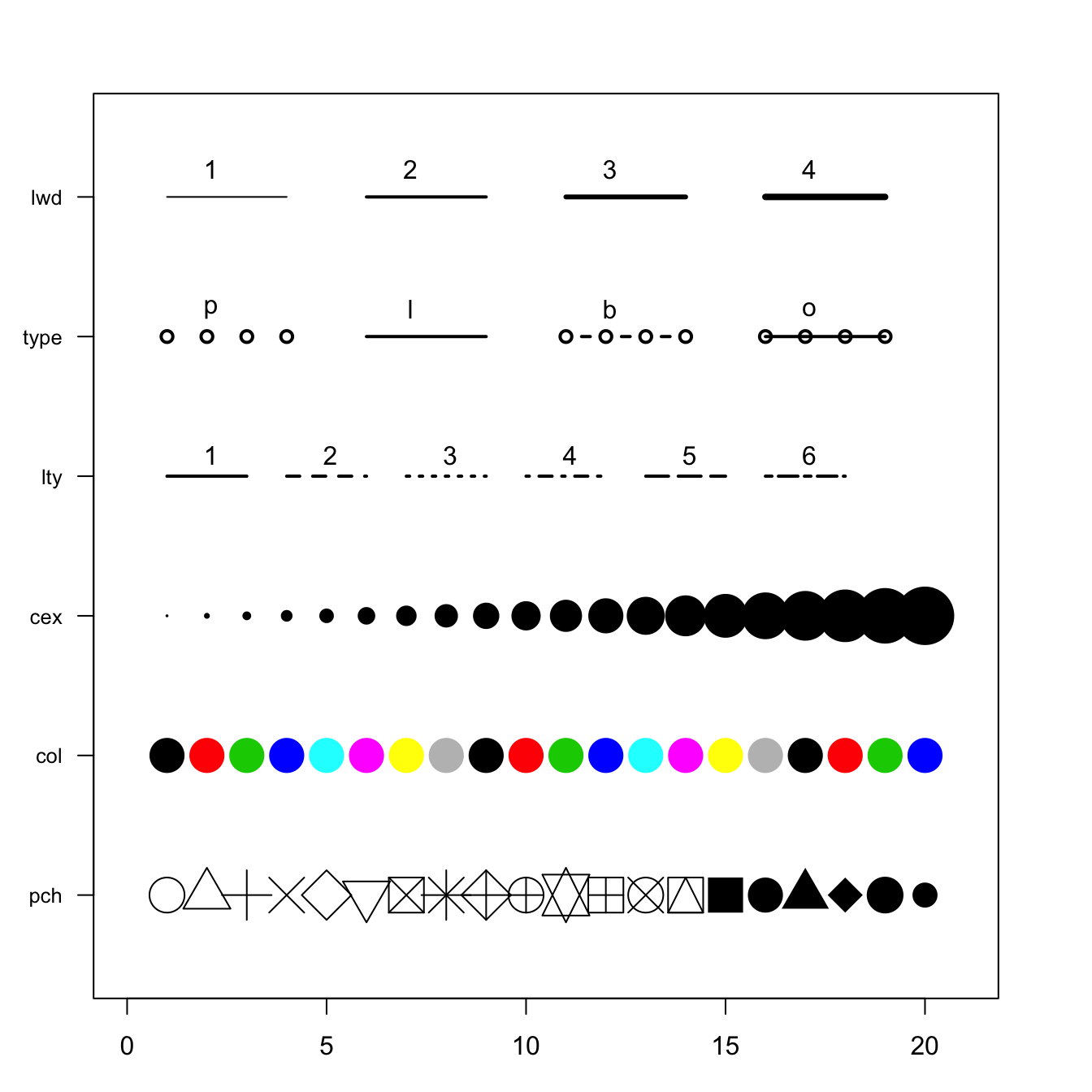# Base R graph parameters: a cheatsheet.

This post aims to remind the options offered to customize a graph in base R. Understand in a sec how to use `lwd`, `pch`, `type`, `lty`, `cex`, and more.

Base R offers many option to customize the chart appearance. Basically everthing is doable with those few options:

• `cex` → shape size
• `lwd` → line width
• `col` → control colors
• `lty` → line type
• `pch` → marker shape
• `type` → link between dots

Note: visit the cheatsheet section for more.``````# initialization
par(mar=c(3,3,3,3))
num <- 0 ;
num1 <- 0
plot(0,0 , xlim=c(0,21) , ylim=c(0.5,6.5), col="white" , yaxt="n" , ylab="" , xlab="")

#fill the graph
for (i in seq(1,20)){
points(i,1 , pch=i , cex=3)
points(i,2 , col=i , pch=16 , cex=3)
points(i,3 , col="black" , pch=16 , cex=i*0.25)

#lty
if(i %in% c(seq(1,18,3))){
num=num+1
points(c(i,i+2), c(4,4) , col="black" , lty=num , type="l" , lwd=2)
text(i+1.1 , 4.15 , num)
}

#type and lwd
if(i %in% c(seq(1,20,5))){
num1=num1+1
points(c(i,i+1,i+2,i+3), c(5,5,5,5) , col="black"  , type=c("p","l","b","o")[num1] , lwd=2)
text(i+1.1 , 5.2 , c("p","l","b","o")[num1] )
points(c(i,i+1,i+2,i+3), c(6,6,6,6) , col="black"  , type="l",  lwd=num1)
text(i+1.1 , 6.2 , num1 )

}
}